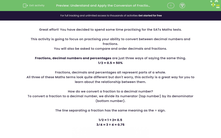# Understand and Apply the Conversion of Fractions to Decimal Numbers 2

In this worksheet, students will be asked to practice the skill of converting decimal numbers to fractions and vice versa. They will also be asked to order and compare decimal numbers and fractions.This content is premium and exclusive to EdPlace subscribers.Key stage:  KS 2

Curriculum topic:   Exam-style Questions: SATs Maths

Curriculum subtopic:   Exam-Style Questions: Decimal Conversion

Difficulty level:#### Worksheet Overview

Great effort! You have decided to spend some time practising for the SATs Maths tests.

This activity is going to focus on practising your ability to convert between decimal numbers and fractions.

You will also be asked to compare and order decimals and fractions.

Fractions, decimal numbers and percentages are just three ways of saying the same thing.

1⁄2 = 0.5 = 50%

Fractions, decimals and percentages all represent parts of a whole.

All three of these Maths terms look quite different but don't worry, this activity is a great way for you to learn about the relationship between them.

How do we convert a fraction to a decimal number?

To convert a fraction to a decimal number, we divide its numerator (top number) by its denominator (bottom number).

The line separating a fraction has the same meaning as the ÷ sign.

1⁄2 = 1 ÷ 2= 0.5

3⁄4 = 3 ÷ 4 = 0.75Remember to use the bus stop method of division to help you solve the calculation.

Over to you now to have a go at some practice questions...good luck!

### What is EdPlace?

We're your National Curriculum aligned online education content provider helping each child succeed in English, maths and science from year 1 to GCSE. With an EdPlace account you’ll be able to track and measure progress, helping each child achieve their best. We build confidence and attainment by personalising each child’s learning at a level that suits them.

Get started